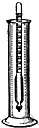acidimetry

Also found in: Thesaurus, Encyclopedia, Wikipedia.
Related to acidimetry: alkalimetry

ac·i·dim·e·ter

(ăs′ĭ-dĭm′ĭ-tər)
n.
A hydrometer used to determine the specific gravity of acid solutions.

ac′i·dim′e·try n.

acidimetry

(ˌæsɪˈdɪmɪtrɪ)
n
(Chemistry) the determination of the amount of acid present in a solution, measured by an acidimeter or by volumetric analysis
Collins English Dictionary – Complete and Unabridged, 12th Edition 2014 © HarperCollins Publishers 1991, 1994, 1998, 2000, 2003, 2006, 2007, 2009, 2011, 2014

acidimetry

Chemistry. the determination of the amount of free acid in a liquid. — acidimeter, n. — acidimetrical, adj.
 Noun 1acidimetry - volumetric analysis using standard solutions of acids to measure the amount of a base presentvolumetric analysis - quantitative analysis by the use of definite volumes of standard solutions or reagents